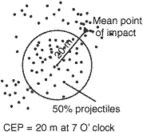# circular error probability

Also found in: Dictionary.

## circular error probability (CEP)The radius of a circle within which half the missiles or projectiles are expected to fall. It is an indicator of the delivery accuracy of a weapon system, used as a factor in determining probable damage to a target. The CEP is expressed as a distance and a clock code from the desired point of impact. Also called circular probable error.
References in periodicals archive ?
Accuracy is measured by what is called CEP (Circular Error Probability), essentially the measured diameter of a circle in which 50 percent of rounds will impact.
In military parlance, that's called 'circular error probability'.
Teseo's GPS accuracy expressed in circular error probability (the radius of a circle that represents a 50% probability of a position lying in that circle) is as low as 2 meters.
Overall, terrain referenced navigation accuracies of 30 m circular error probability (horizontal) and 3 m linear error probability (vertical) are reported as being achievable over terrain rougnesses down to two per cent.
In less than a minute, the position of a downed airman can be determined to within a 15-m circular error probability using the precise positioning service GPS mode.

Site: Follow: Share:
Open / Close